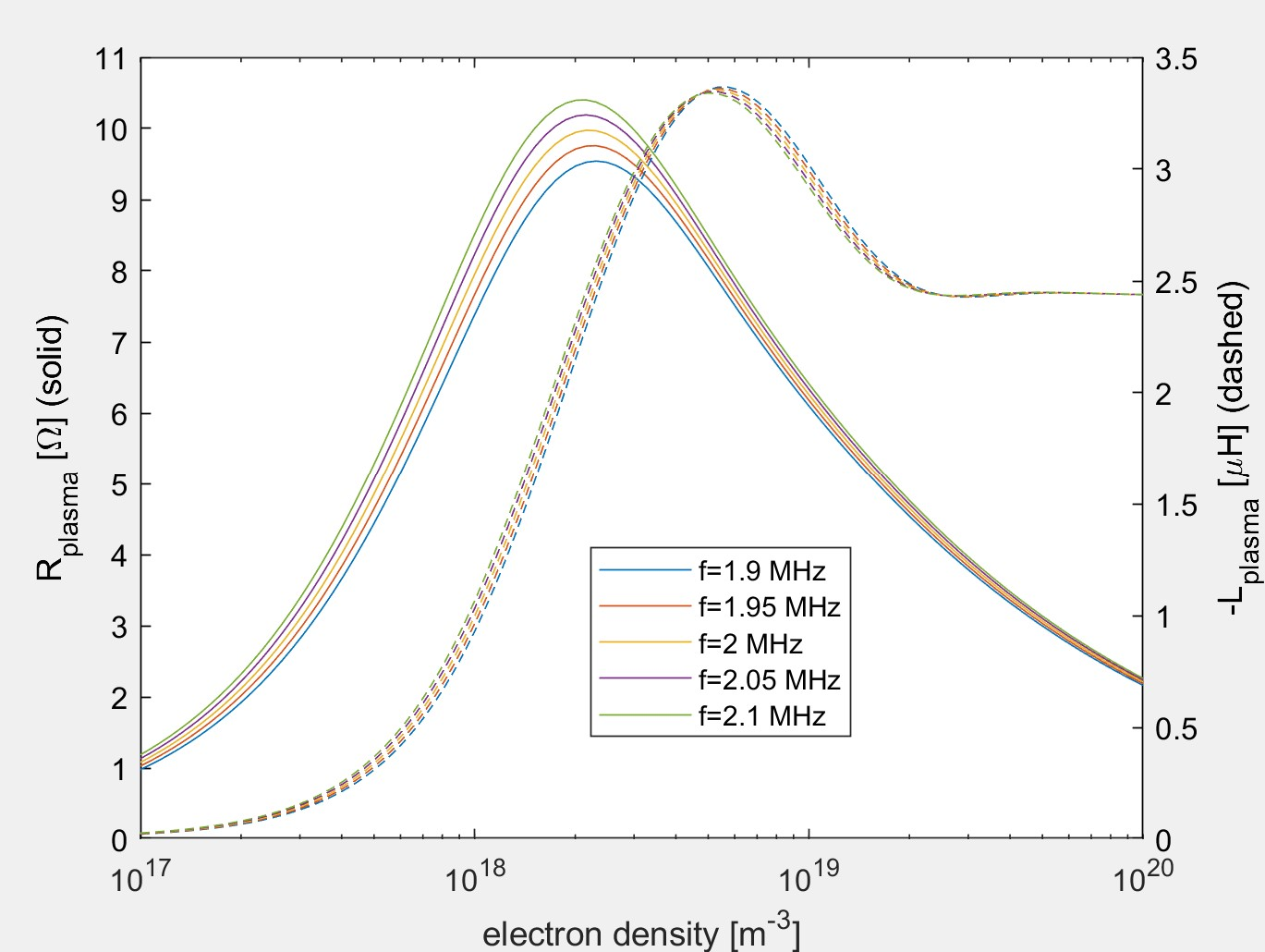# Plasma Circuit Models for RF Heating

This summer, I worked on creating a plasma circuit model as part of PSS’s work under the ARPA-E GAMOW grant. As part of this project, I wrote MATLAB functions to reproduce the results of two papers on impedance of radio frequency (RF)-driven circuits for plasma heating. Both functions take in some plasma and geometric parameters and return impedance values as well as plots of impedance as a function of other parameters.

The first function is based on reference , which uses the transformer model to describe the coupling between the plasma and the rest of the circuit. This means that the plasma can be represented as a resistor-inductor circuit that is inductively coupled with the main circuit. In addition to calculating the equivalent circuit impedance for values in passed-in density and frequency ranges, I reproduced the figures showing resistance/inductance and reflection coefficient as a function of the electron density of the plasma. Plotting over many orders of magnitude of density, you can see drastic changes in the plasma resistance and inductance.Plasma resistance (solid lines) and inductance (dashed lines) as a function of electron density for five different frequencies, based on the transformer model in .

Since ion cyclotron resonance heating (ICRH) is a leading technique for plasma heating in fusion reactors, we also wanted a function that dealt specifically with ICRH in its plasma model. I then read through some literature on ICRH in order to find a suitable reference to model in MATLAB. I found this in reference , which became the basis for my second function. This model uses transmission line theory to calculate the antenna impedance with the effects of the plasma incorporated. This paper also compared a previously-formulated 2D model with its own 3D model, and the implications of this extension to three dimensions can be seen in the way impedance changes as a function of the wavenumber.Antenna reactance (left) and resistance (right) as a function of the parallel wavenumber, based on the transmission line model in .

The impedance values produced from both of these models can be used to help account for the effect of plasma on antennas used in RF heating, especially ICRH. While assumptions are made in these models to allow for analytical calculations to be made (notably assuming uniform current density and neglecting volume propagation effects) and adjustments are needed to resolve minor discrepancies between the MATLAB models and the figures in the reference papers, they should be a reasonable first approximation of the physics that is occurring and the impedance generated by the plasma.

This summer has given me a lot more knowledge about plasma physics, in particular about resonance heating. I have also gained a lot of experience in conducting literature reviews, reproducing published results, and working in MATLAB.

 Nishida, K., et al., “Equivalent circuit of radio frequency-plasma with the transformer model.” Rev. Sci. Instrum. 85, 02B117 (2014); https://doi.org/10.1063/1.4832060.

 Bhatnagar, V.P., et al., “A 3-D analysis of the coupling characteristics of ion cyclotron resonance heating antennae.” Nucl. Fusion 22, 280 (1982); https://doi.org/10.1088/0029-5515/22/2/011.

This site uses Akismet to reduce spam. Learn how your comment data is processed.